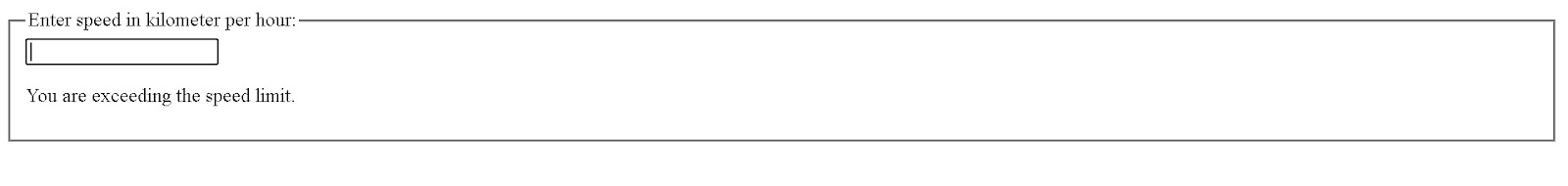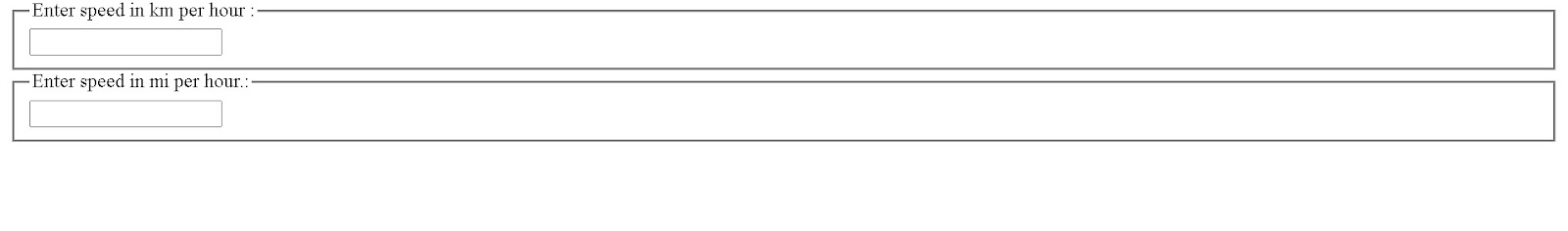# Lifting The State Up in ReactJS

## Introduction

In React, each component has its state. Lifting the state up is a valuable concept for React developers since we often have a state that is accommodated within a single component but needs to be shared with its siblings. We lift the state up to make the parent state a single shared state and a sole "source of truth" and pass the parent's data to its children.

This concept is called lifting state up. It is of great use to maintain data consistency in our react applications.

## When to lift the state up?

So when to do the process of lifting the state up?. Many times, multiple components must reflect the same changing data. And if the data is not in sync between the "parent and children components" or "cousin components", it is recommended to lift the shared state up to the closest common ancestor.

Let's see how it works in actual practice.

Here, we'll make a speed calculator to see if we're within the speed limit.

On the output page, it will display three sections:

1. Accepting the speed input in kilometers per hour (kmph)
2. Receiving speed input in miles per hour
3. The line will tell you whether you are within the speed limit.

In addition, we want our speeds from both inputs to be synced. The change in one should be reflected by the other too.

Let's see how we do it step by step:

First, we'll create a component called SpeedMonitor that accepts the 'kmph' speed in  kilometers per hour as a prop and displays whether it's within the limit:

Code:

``````function SpeedMonitor(props) {
if (props.kmph <= 80) {
return <p>You are within the speed limit.</p>;
}

return <p>You are exceeding the speed limit.</p>;
}
``````

After that, we'll make a component called Calculator. It creates an <input> element that allows you to enter the speed and saves its value in this.state.speed.

In addition, the SpeedMonitor is rendered for the current input value.

Code:

``````class Calculator extends React.Component {
constructor(props) {
super(props);
this.handleChange = this.handleChange.bind(this);
this.state = {speed: ''};
}

handleChange(e) {
this.setState({speed: e.target.value});
}

render() {
const speed = this.state.speed;

return (
<fieldset>
<legend>Enter speed in kilometer per hour:</legend>
<input
value={speed}
onChange={this.handleChange} />
<SpeedMonitor
kmph={parseFloat(speed)} />
</fieldset>
);
}
}``````Output

## Taking a second input

Now our next aim is to take a second input in miles per hour in addition to our previous input in kilometers per hour, and we even want them to be in sync.

To begin, we will extract a SpeedInput component from the Calculator. We will add a new scale prop to it that can be either "km" or "mi":

``````Code:
const scaleNames = {
km: 'km per hour ',
mi: 'mi per hour.'
};

class SpeedInput extends React.Component {
constructor(props) {
super(props);
this.handleChange = this.handleChange.bind(this);
this.state = {speed: ''};
}

handleChange(e) {
this.setState({speed: e.target.value});
}

render() {
const speed = this.state.speed;
const scale = this.props.scale;

return (
<fieldset>
<legend>Enter speed in {scaleNames[scale]}:</legend>
<input value={speed}
onChange={this.handleChange} />
</fieldset>
);
}
}``````

We can now use the Calculator to display two different speed inputs:

Code:

``````class Calculator extends React.Component {
render() {
return (
<div>
<SpeedInput scale="km" />
<SpeedInput scale="mi" />
</div>
);
}
}
``````Output

Now we have two inputs, but when you change the speed in one of them, the other does not change. At this stage, they are not in sync.

The Calculator's SpeedMonitor is also unavailable for display. This is because the current speed is hidden inside the SpeedInput, and the Calculator doesn't know it.

## Writing conversions

Let's write two functions to convert KMPH to MPH and vice versa.

Code:

``````function toKmph(miph) {
return (miph / 0.6213);
}

function toMiph(kmph) {
return (kmph * 0.6213);
}``````

Both of these functions convert numbers. We'll create a new function that takes two arguments: a string speed and a converter function, and returns a string. We'll use it to determine the value of one input based on the value of the other.

It returns an empty string when the speed is invalid, and the output is rounded to the third decimal place:

Code:

``````function tryConvert(speed, convert) {
const input = parseFloat(speed);

if (Number.isNaN(input)) {
return '';
}

const output = convert(input);
const rounded = Math.round(output * 1000) / 1000;

return rounded.toString();
}``````

## Lifting the state up

At the current state, both the SpeedInput components keep their values independently. But we want them to be in sync with each other. The change in KMPH should reflect the converted change in MPH and vice versa.

Sharing state in React is done by pushing it up to the nearest common ancestor of the components that require it. This is referred to as "raising state up." The local state will be removed from the SpeedInput and moved to the Calculator instead.

Since the Calculator owns the shared state, it becomes the "source of truth" for the current speed in both inputs. It can instruct the child components to have values that are in sync with one another. The two inputs will always be in sync because the props of both SpeedInput components come from the same parent Calculator component.

Code:

``````class SpeedInput extends React.Component {
constructor(props) {
super(props);
this.handleChange = this.handleChange.bind(this);
}

handleChange(e) {
this.props.onSpeedChange(e.target.value);
}

render() {
const speed = this.props.speed;
const scale = this.props.scale;

return (
<fieldset>
<legend>Enter speed in {scaleNames[scale]}:</legend>
<input value={speed}
onChange={this.handleChange} />
</fieldset>
);
}
}``````

Let's see this happening step by step.

In the SpeedInput component, we'll first change this.state.speed with this.props.speed. Let's suppose this.props.speed already exists for the time being, even though we'll need to pass it from the Calculator later:

Code:

``````render() {
// Before: const speed = this.state.speed;

const speed = this.props.speed;
// ...``````

As we know, props are read-only. The SpeedInput could call this.setState when the speed was in the local state. On the other hand, the SpeedInput has no control over the speed because it comes from the parent as a prop.

This is commonly fixed in React by making a component "controlled." The custom SpeedInput can accept both speed and onSpeedChange props from its parent Calculator.

Now SpeedInput calls this.props.onSpeedChange: when it needs to update its speed.

Code:

``````handleChange(e) {
// Before: this.setState({speed: e.target.value});

this.props.onSpeedChange(e.target.value);
// ...
``````

The parent Calculator component will provide the onSpeedChange prop with the speed prop.

It will respond to the change by changing its local state, causing both inputs to be re-rendered with the new values.

Let's recollect the changes we have made to the SpeedInput so far. We removed the local state from it, and now we are reading this.props.speed instead of this.state.speed. We now call this.props.onSpeedChange(), instead of calling this.setState(), which will be provided by the Calculator whenever we want to make a change.

``````Code:
class SpeedInput extends React.Component {
constructor(props) {
super(props);
this.handleChange = this.handleChange.bind(this);
}

handleChange(e) {``````

Let's move on to the Calculator component.

We'll save the speed and scale of the current input in its local state. This is the "source of truth" for both of them, as it is the state we "lifted up" from the inputs. It's the simplest representation of all the information we need to render both inputs.

We could have saved the values of both inputs, but that would have been wasteful. It is sufficient to save the value of the most recently updated input and the scale it reflects. The value of the other input can then be inferred solely based on the current speed and scale.

Now the inputs stay in sync because their value is calculated from derived from the same state.

Now, no matter which input you edit, this.state.speed and this.state.scale in the Calculator get updated. The value of one input will be recalculated when the value of the other is changed.

Code:

``````class Calculator extends React.Component {
constructor(props) {
super(props);
this.handleKmphChange = this.handleKmphChange.bind(this);
this.handleMiphChange = this.handleMiphChange.bind(this);
this.state = {speed: '', scale: 'km'};
}

handleKmphChang(speed) {
this.setState({scale: 'km', speed});
}

handleMiphChange(speed) {
this.setState({scale: 'mi', speed});
}

render() {
const scale = this.state.scale;
const speed = this.state.speed;
const kmph = scale === 'mi' ? tryConvert(speed, toKmph) : speed;
const miph = scale === 'km' ? tryConvert(speed, toMiph) : speed;

return (
<div>
<SpeedInput
scale="km"
speed={kmph}
onSpeedChange={this.handleKmphChange} />
<SpeedInput
scale="mi"
speed={miph}
onSpeedChange={this.handleKmphChange} />
<SpeedMonitor
kmph={parseFloat(kmph)} />
</div>
);
}
}``````Output

1. What does lifting the state up mean?

Ans:- In React, whenever a state needs to be shared with siblings. We lift the state up to the closest common ancestor to make it a sole source of truth. This is called "Lifting The State Up."

2. When should we do the process of lifting the state up?

Ans:- Many times, multiple components must reflect the same changing data. And if the data is not in sync between the "parent and children components" or "cousin components," it is recommended to lift the shared state up to the closest common ancestor.

3. What is the state in React?

Ans:- React uses a simple JavaScript object called the state to represent information about the current state of a component. Since the state is dynamic, it allows a component to maintain track of changing data between renderings while remaining dynamic and interactive.

## Key takeaways

It's better to have a single "source of truth" for any changing data in our react application. We often have a state contained within a single component but needs to be shared with siblings. In such cases, we can use the lifting of state up.

Rather than using a full-fledged state management framework like Redux or React Context, we can lift the state up to the next common ancestor and transmit the state variables, state values, and any callbacks to change that state down. Using this method also reduces bugs' surface area and makes it easier to implement any custom logic.

Want to learn more about React JS?, check out this course, Advanced Front End Web Development Course — React.js offered by Coding Ninjas, and learn to build interactive web pages!. Practice fifteen most frequently asked React JS interview questions here